# Nonlinear Heat Transfer in Thin Plate

This example shows how to perform a heat transfer analysis of a thin plate.

The plate is square and the temperature is fixed along the bottom edge. No heat is transferred from the other three edges (i.e. they are insulated). Heat is transferred from both the top and bottom faces of the plate by convection and radiation. Because radiation is included, the problem is nonlinear. One of the purposes of this example is to show how to handle nonlinearities in PDE problems.

Both a steady state and a transient analysis are performed. In a steady state analysis we are interested in the final temperature at different points in the plate after it has reached an equilibrium state. In a transient analysis we are interested in the temperature in the plate as a function of time. One question that can be answered by this transient analysis is how long does it take for the plate to reach an equilibrium temperature.

### Heat Transfer Equations for the Plate

The plate has planar dimensions one meter by one meter and is 1 cm thick. Because the plate is relatively thin compared with the planar dimensions, the temperature can be assumed constant in the thickness direction; the resulting problem is 2D.

Convection and radiation heat transfer are assumed to take place between the two faces of the plate and a specified ambient temperature.

The amount of heat transferred from each plate face per unit area due to convection is defined as

`${Q}_{c}={h}_{c}\left(T-{T}_{a}\right)$`

where ${T}_{a}$ is the ambient temperature, $T$ is the temperature at a particular x and y location on the plate surface, and ${h}_{c}$ is a specified convection coefficient.

The amount of heat transferred from each plate face per unit area due to radiation is defined as

`${Q}_{r}=ϵ\sigma \left({T}^{4}-{T}_{a}^{4}\right)$`

where $ϵ$ is the emissivity of the face and $\sigma$ is the Stefan-Boltzmann constant. Because the heat transferred due to radiation is proportional to the fourth power of the surface temperature, the problem is nonlinear.

The PDE describing the temperature in this thin plate is

`$\rho {C}_{p}{t}_{z}\frac{\partial T}{\partial t}-k{t}_{z}{\nabla }^{2}T+2{Q}_{c}+2{Q}_{r}=0$`

where $\rho$ is the material density, ${C}_{p}$ is the specific heat, ${t}_{z}$ is the plate thickness, and the factors of two account for the heat transfer from both plate faces.

It is convenient to rewrite this equation in the form expected by PDE Toolbox

`$\rho {C}_{p}{t}_{z}\frac{\partial T}{\partial t}-k{t}_{z}{\nabla }^{2}T+2{h}_{c}T+2ϵ\sigma {T}^{4}=2{h}_{c}{T}_{a}+2ϵ\sigma {T}_{a}^{4}$`

### Problem Setup

The plate is composed of copper which has the following properties:

```k = 400; % thermal conductivity of copper, W/(m-K) rho = 8960; % density of copper, kg/m^3 specificHeat = 386; % specific heat of copper, J/(kg-K) thick = .01; % plate thickness in meters stefanBoltz = 5.670373e-8; % Stefan-Boltzmann constant, W/(m^2-K^4) hCoeff = 1; % Convection coefficient, W/(m^2-K) % The ambient temperature is assumed to be 300 degrees-Kelvin. ta = 300; emiss = .5; % emissivity of the plate surface```

Create the PDE model with a single dependent variable.

```numberOfPDE = 1; model = createpde(numberOfPDE);```

For a square, the geometry and mesh are easily defined as shown below.

```width = 1; height = 1;```

Define the square by giving the 4 x-locations followed by the 4 y-locations of the corners.

```gdm = [3 4 0 width width 0 0 0 height height]'; g = decsg(gdm, 'S1', ('S1')');```

Convert the DECSG geometry into a geometry object on doing so it is appended to the PDEModel

`geometryFromEdges(model,g);`

Plot the geometry and display the edge labels for use in the boundary condition definition.

```figure; pdegplot(model,'EdgeLabels','on'); axis([-.1 1.1 -.1 1.1]); title 'Geometry With Edge Labels Displayed';```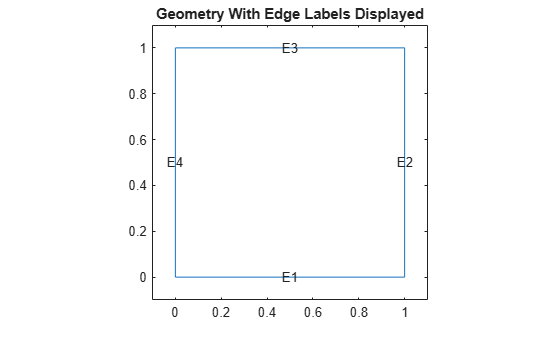Specify the coefficients. The expressions for the coefficients required by PDE Toolbox can easily be identified by comparing the equation above with the scalar parabolic equation in the PDE Toolbox documentation.

`c = thick*k;`

Because of the radiation boundary condition, the "a" coefficient is a function of the temperature, u. It is defined as a MATLAB expression so it can be evaluated for different values of u during the analysis.

```a = @(~,state) 2*hCoeff + 2*emiss*stefanBoltz*state.u.^3; f = 2*hCoeff*ta + 2*emiss*stefanBoltz*ta^4; d = thick*rho*specificHeat; specifyCoefficients(model,'m',0,'d',0,'c',c,'a',a,'f',f);```

The bottom edge of the plate is set to 1000 degrees-Kelvin.

Apply the boundary conditions. Three of the plate edges are insulated. Because a Neumann boundary condition equal zero is the default in the finite element formulation, the boundary conditions on these edges do not need to be set explicitly. A Dirichlet condition is set on all nodes on the bottom edge, edge 1,

`applyBoundaryCondition(model,'dirichlet','Edge',1,'u',1000);`

Specify the initial guess.

`setInitialConditions(model,0);`

Create the triangular mesh on the square with approximately ten elements in each direction.

```hmax = .1; % element size msh = generateMesh(model,'Hmax',hmax); figure; pdeplot(model); axis equal title 'Plate With Triangular Element Mesh' xlabel 'X-coordinate, meters' ylabel 'Y-coordinate, meters'```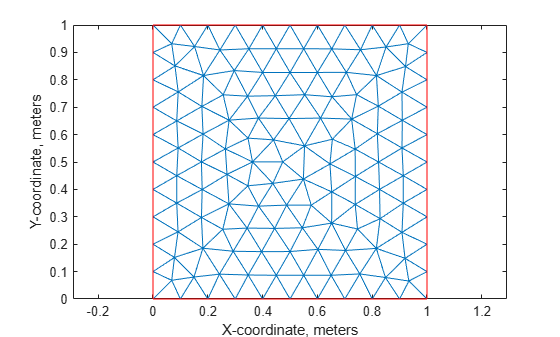Because the a and f coefficients are functions of temperature (due to the radiation boundary conditions), `solvepde` automatically picks the nonlinear solver to obtain the solution.

```R = solvepde(model); u = R.NodalSolution; figure; pdeplot(model,'XYData',u,'Contour','on','ColorMap','jet'); title 'Temperature In The Plate, Steady State Solution' xlabel 'X-coordinate, meters' ylabel 'Y-coordinate, meters' axis equal```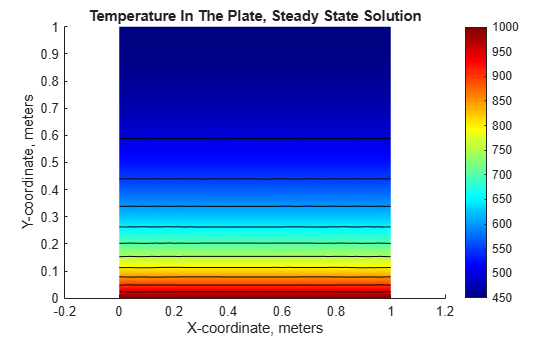```p = msh.Nodes; plotAlongY(p,u,0); title 'Temperature As a Function of the Y-Coordinate' xlabel 'Y-coordinate, meters' ylabel 'Temperature, degrees-Kelvin'```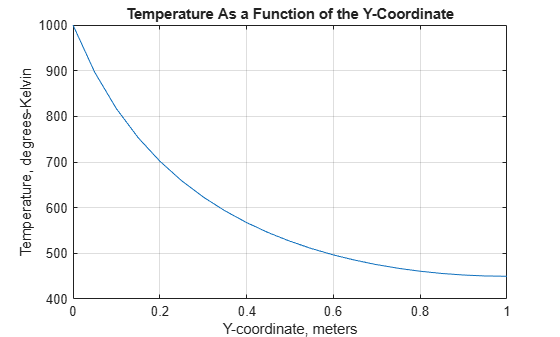```fprintf('Temperature at the top edge of the plate = %5.1f degrees-K\n', ... u(4));```
```Temperature at the top edge of the plate = 449.8 degrees-K ```

### Transient Solution

Include the `d` coefficient.

```specifyCoefficients(model,'m',0,'d',d,'c',c,'a',a,'f',f); endTime = 5000; tlist = 0:50:endTime; numNodes = size(p,2);```

Set the initial temperature of all nodes to ambient, 300 K.

`u0(1:numNodes) = 300;`

Set the initial temperature on the bottom edge E1 to the value of the constant BC, 1000 K.

`setInitialConditions(model,1000,'edge',1);`

Set the following solver options.

```model.SolverOptions.RelativeTolerance = 1.0e-3; model.SolverOptions.AbsoluteTolerance = 1.0e-4;```

Solve the problem by using `solvepde`. The solver automatically picks the parabolic solver to obtain the solution.

```R = solvepde(model,tlist); u = R.NodalSolution; figure; plot(tlist,u(3, :)); grid on title 'Temperature Along the Top Edge of the Plate as a Function of Time' xlabel 'Time, seconds' ylabel 'Temperature, degrees-Kelvin'```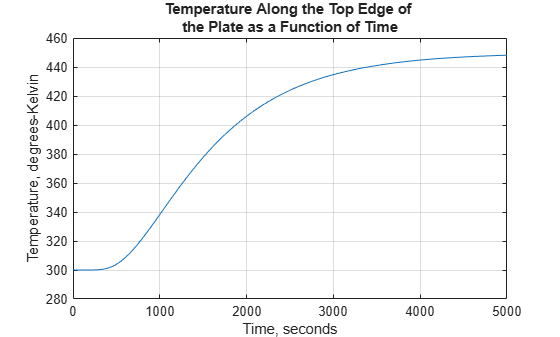```figure; pdeplot(model,'XYData',u(:,end),'Contour','on','ColorMap','jet'); title(sprintf('Temperature In The Plate, Transient Solution( %d seconds)\n', ... tlist(1,end))); xlabel 'X-coordinate, meters' ylabel 'Y-coordinate, meters' axis equal;```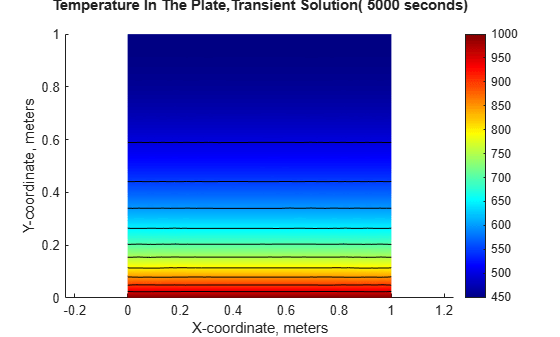```fprintf('\nTemperature at the top edge(t=%5.1f secs)=%5.1f degrees-K\n', ... tlist(1,end), u(4,end));```
```Temperature at the top edge(t=5000.0 secs)=441.8 degrees-K ```

### Summary

The plots of temperature in the plate from the steady state and transient solution at the ending time are very close. That is, after around 5000 seconds, the transient solution has reached the steady state values. The temperatures from the two solutions at the top edge of the plate agree to within one percent.

##### SupportGet trial now